Forward voltage of a diode connected to Vin through a resistor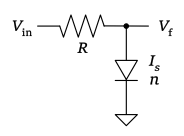#### Vf calculator

 Vin : Input voltage [V] Rs : Series resistance [Ω] Tj : Junction temperature [°C] Diode model Specify 1SS193 BAV70HY BAV70 1N4148 MMBD770 MMBD301 1SS154 BAR43 HSMS-8101 RB400D 1N5819HW SFH4045N SML-D12U8W SML-D12D8W SML-D12W8W SML-D12M8W SML-D12FW SMLD12EN1W SMLD12BN1W TNOM : Nominal temperature [°C] Is(TNOM) : Saturation current [A] n : Emission coefficient Eg : Energy gap [eV] XTI : Is temperature exponent Vf : Forward voltage ≈ [V] If : Forward current ≈ [mA]

Note:
The calculation model used here does not take into account the backward diode and ohmic resistance components.
In the high current range exceeding several mA, the results may differ significantly from the calculations.

#### Formulas used

Eg : energy gap 1.11 [eV]
k : Boltzmann constant 1.380649 × 10-23 [J K-1]
q : elementary charge 1.602176634 × 10-19 [C]
n : emission coefficient
T0 : Nominal temperature in Kelvin. 273.15 + 27 [K]
T1 : Junction temperature in Kelvin. 273.15 + Tj [K]
XTI : Saturation current temperature exponent. Usually equal to 3 for junction diodes, 2 for Schottky barrier diodes.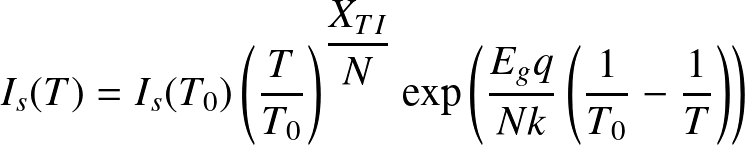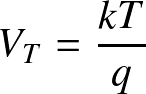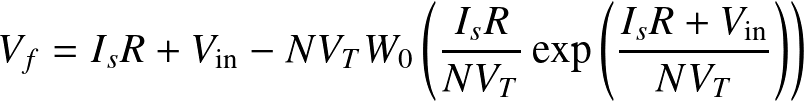For high Vin, such as Vin > 220 n VT, the following Newton-Raphson method is used.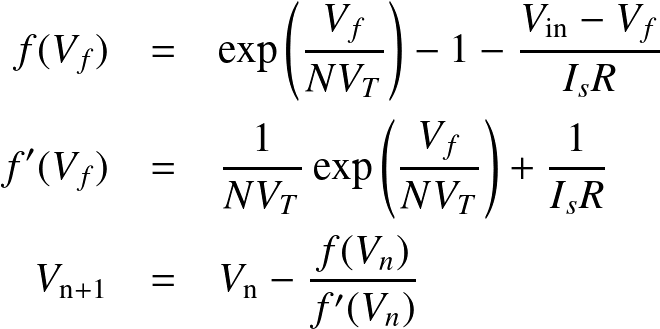#### Appendix

fig.1 Example of a plot of calculation results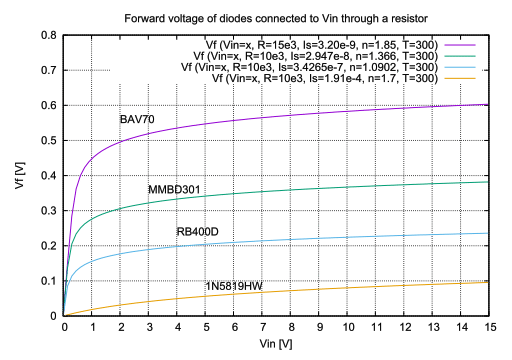fig.2 Example of Vf characteristics of a real diode (Adapted from Nexperia, BAV70 datasheet)
Forward current as a function of forward voltage; typcal values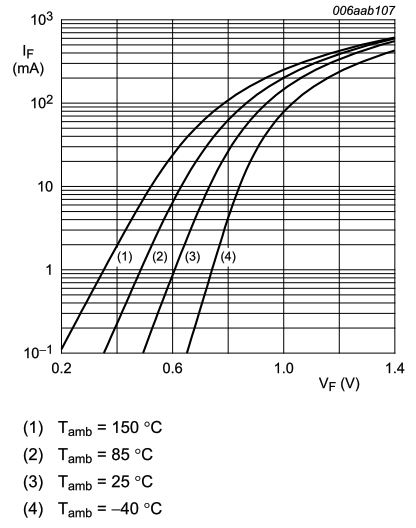#### Reference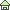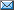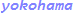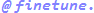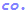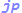© 2000 Takayuki HOSODA.